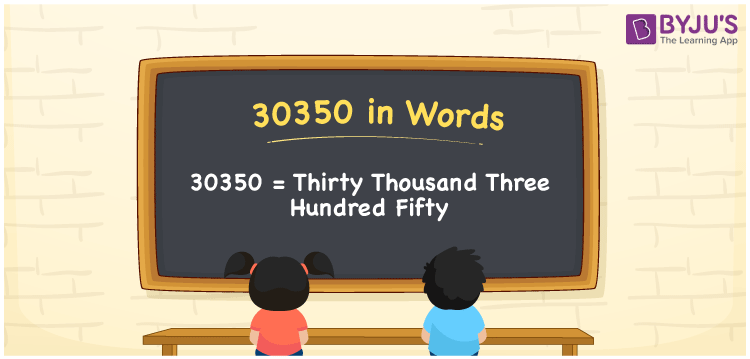# 30350 in Words

We can write 30350 in words as Thirty thousand three hundred fifty. Thus, whenever we come across the number 30350, we can read it as Thirty thousand three hundred fifty. This word form can be derived with the help of a place value chart. In this article, you can learn the easiest method of writing the cardinal number 30350 in words.

 30350 in words Thirty thousand three hundred fifty Thirty thousand three hundred fifty in Numbers 30350

## 30350 in English words

Generally, we can write numbers in words using the English alphabet. So, we can spell 30350 in English as “Thirty thousand three hundred fifty”.## How to Write 30350 in Words?

The below five-column table represents the place value chart for the number 30350.

 Ten thousands Thousands Hundreds Tens Ones 3 0 3 5 0

From the above table, we can expand the digits with respect to their place values as:

3 × Ten thousand + 0 × Thousand + 3 × Hundred + 5 × Ten + 0 × One

= 3 × 10000 + 0 × 1000 + 3 × 100 + 5 × 10 + 0 × 1

= 30000 + 300 + 50

= Thirty thousand + Three hundred + Fifty

= Thirty thousand three hundred fifty

Therefore, 30350 in words = Thirty thousand three hundred fifty.

### Facts About the Number 30350

30350 is a natural number that is the successor of 30349 and the predecessor of 30351.

30350 in words – Thirty thousand three hundred fifty

Is 30350 an odd number? – No

Is 30350 an even number? – Yes

Is 30350 a perfect square number? – No

Is 30350 a perfect cube number? – No

Is 30350 a prime number? – No

Is 30350 a composite number? – Yes

## Frequently Asked Questions on 30350 in Words

Q1

### How do you express 30350 in words?

We can express the number 30350 in words as Thirty thousand three hundred fifty.
Q2

### How do you write Rs. 30350 in words on a cheque?

On a cheque, an amount of 30350 can be written in words as “Thirty thousand three hundred fifty rupees only”.
Q3

### Find the value of Thirty thousand three hundred fifty plus One thousand one hundred fifty.

Thirty thousand three hundred fifty plus One thousand one hundred fifty = 30350 + 1150 = 31500